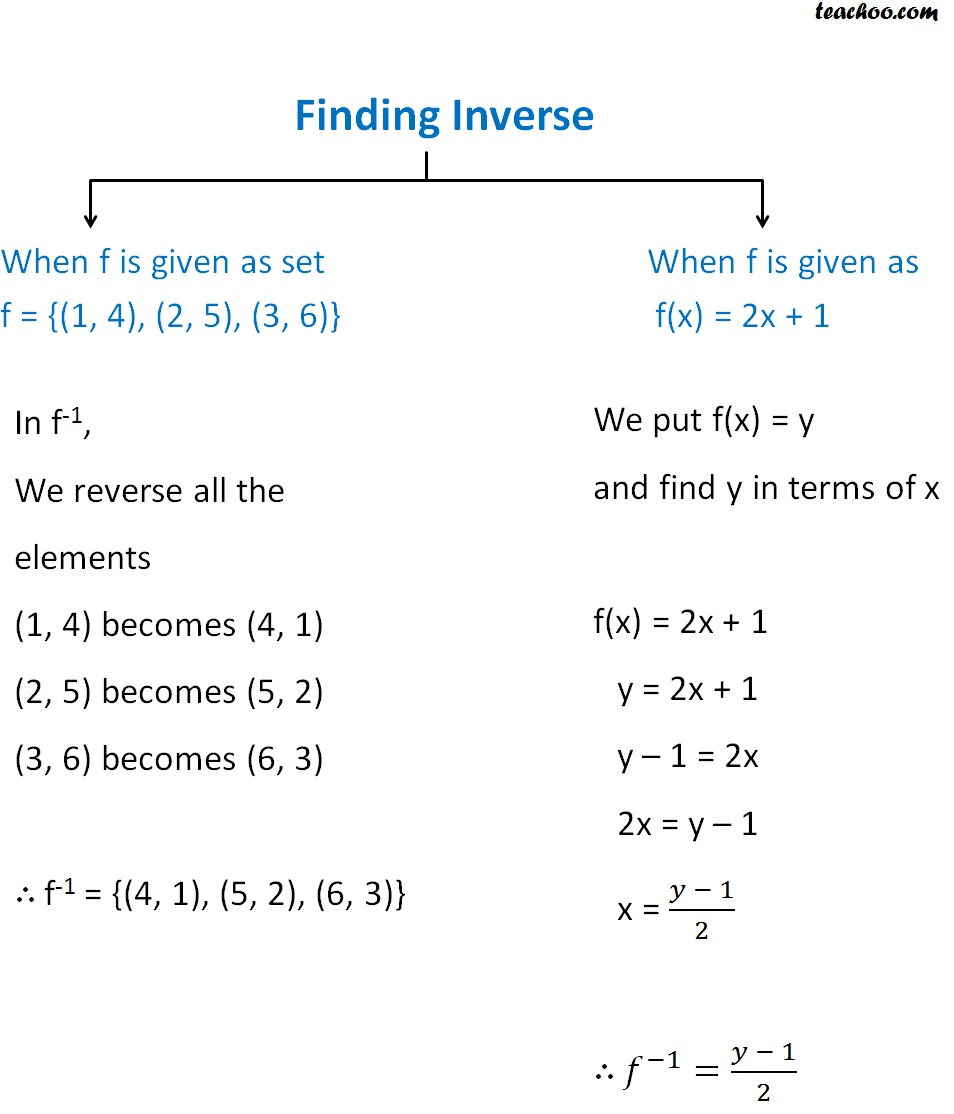Finding Inverse

Chapter 1 Class 12 Relation and Functions
Concept wise

We find inverse in two scenarios

1. When f is given as set
2. When f is given as a function

#### f = {(1, 4), (2, 5), (3, 6)}

In f -1 ,

We reverse all the elements

(1, 4) becomes (4, 1)

(2, 5) becomes (5, 2)

(3, 6) becomes (6, 3)

∴ f -1 = {(4, 1), (5, 2), (6, 3)}

#### f(x) = 2x + 1

We put f(x) = y

and find y in terms of x

f(x) = 2x + 1

y = 2x + 1

y – 1 = 2x

2x = y – 1

x = (y - 1)/2

∴ f -1  = (y - 1)/2

To summariseLearn in your speed, with individual attention - Teachoo Maths 1-on-1 Class